Rotating Terrains using Functions

Friday, March 07th, 2008 | Author:

A while ago, someone asked me how to rotate heightfields. Not even every terrain editor offers an option to rotate a heightfield 90°, which could be easily implemented. TG2 doesn’t provide this functionality either, let alone rotation by an arbitrary angle. Only 180° rotation is possible – by setting both Flip options.

Using the following method, you will be able to rotate heightfields by an angle in the range (-90°, 90°). That is excluding -90° and 90°. Rotations by 90° and multiples should be performed in an external editor.

Idea und Implementation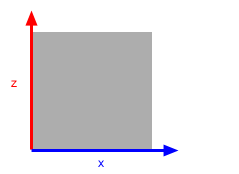The idea is as follows: We can not assign a certain point to a new position just like that, but we can calculate the theoretical position after rotation and try to move the point there. Unfortunately, you can’t really do this in TG2. Which is why we’ll take a different approach.
In the illustrations on the right the z and x axes are plotted as well as a grey square. Those drawings show what we want to do. In addition, there is a screenshot of the node network below, showing the implementation of the rotation in detail.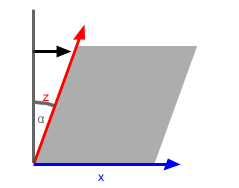Step 1: The higher the z value of a point, the further it has to be moved along the x vector. To achieve this, we use Displacement (Lateral Only), which we restrict to the x vector using the Redirect shader. Thanks to Get Position in Geometry, the axis is being displaced, too.
The alpha (α) angle is the angle by which we rotate the shader. The method to calculate the right factor for displacement from Alpha will be explained below.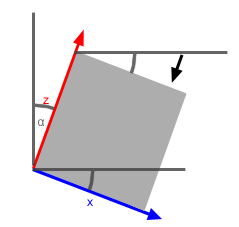Step 2: The higher the x value, the more displacement along the negative z vector. Instead of inverting the scalar, you can set the Multiplier in the Displacement Shader to -1, this saves a Shader and thus render time.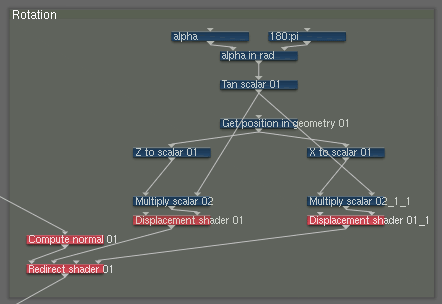Factor and Implementation Details

The higher the z value of a point, the further it is moved along the x vector. The question now is: If z is, say 1m – do we move that point by 1m too? That depends on the angle by which we rotate, if it’s greater then ratio of z and the Displacement Strength is greater too. That ratio is exactly the tangent of our angle Alpha. (Explanations for tangent etc. can be found at Wikipedia)

Luckily, TG2 is already equipped with the tangent function. However, it doesn’t take values in degrees but in radian, instead. It is: 180°=π(pi) rad. As I want to enter the Alpha scalar in degrees, I have to convert the degree value by division by 180°/π. (You could also multiply by π/ 180°)

All we have to do now is multiply the z and x values by the above calculated ratio, push them through a Displacement Shader and hook them up to a Redirect Shader. A single Redirect Shader is enough.

Application and Side Effects

The method described is working quite well. The following images show (from left to right): The terrain without any rotation, the terrain rotated by 0°, 45°, -45°. Camera, sun and terrain scale have been adjusted each time for comparability.

There’s no visible difference between rotation by 0° and disabled rotation. The terrain doesn’t change using angles of ±45° either. Slight differences in the look of the surface are caused by the shaders used for Fractal Breakup, which were not rotated.

This is what a rotation by 45° looks like in the preview: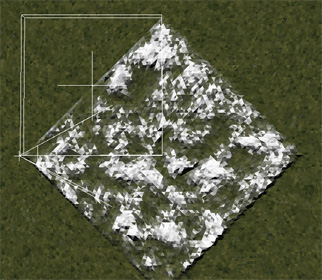It’s easy to see that the heightfield is much wider now. By the factor 1/cos(α) wider. You can use a Heightfield-Resize-Operator to scale the width and length of the heightfield down to original width · cos(α). You have to calculate this manually, Google’s built-in calculator will help you with that. Heightfield-Resize also resizes height – in order to undo this, we multiply the height by 1/cos(α) using Adjust Vertical or the Height-Multiplier in the Heightfield Shader.

That procedure gets more important as the angles get larger. However, values of 70° or higher will cause heavy side effects like strong darkening of the terrain and other by-products of extreme displacement. Therefore, when trying to rotate by large angles you should rotate in 90° steps in an external program beforehand.

Clip File and Usage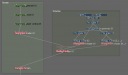The clip file can be downloaded here. The screen shot on the right shows how to integrate it with your network.

That’s it! I hope I explained this method clearly.
Comments and critique are welcome, as always.Tags »